## 30 Nov 2008

### presentation of Edwards & Penney's work, by by Corry Shores[Search Blog Here. Index-tags are found on the bottom of the left column.][Central Entry Directory][Mathematics, Calculus, Geometry, Entry Directory][Calculus Entry Directory][Edwards & Penney, Entry Directory]

Edwards & Penney's Calculus is an incredibly-impressive, comprehensive, and understandable book. I highly recommend it.

An infinite series is an infinite sequence whose terms are summed, and it takes the form: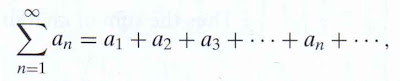here, {an} is an infinite sequence of real numbers, and the number an is the nth term of the series. We may abbreviate the left side of the equation with:

We will now consider the sums of infinite sequences, for example:

We cannot easily sum an infinity of terms, but we may add a finite sequence, so the sum of the first five terms of this sequence is:

We could continue adding five more terms at a time, and we see how this progresses:

We see that the series appears to get closer and closer to 1 as we continually add more terms. Thus we might say that the sum of the whole infinite series is 1:

But we may at least determine sums of a finite part of an infinite sequence, that is, to find partial sums of it.

Thus we may say that infinite series are made up not merely of infinite sequences of terms, but as well of infinite sequences of partial sums:

and so on. The sum of an infinite series is defined as the limit of its sequence of partial sums, so long as it actually has such a limit.

exists (and is finite). Otherwise we say that the series diverges (or is divergent). If a series diverges, then it has no sum.

Thus, so long as it has a limit, the sum of an infinite series is a limit of finite sums:

For example, we might want to find the sum of -- and show that there is a convergence in -- the series:

The first four partial sums of this series are:

We see that in each case, the numerator is n-squared minus 1, over n-squared, so it seem probable that

In fact, we know that this is so by induction:

For this reason, the sum of our series is:

We arrive at the middle equation by setting 2^n over 2^n, and negative 1 over 2^n. Then as 1/2^n goes to infinity, it becomes 0, hence to subtract it from 1 leaves 1. We see below a graph of the partial sums of this series:

from Edwards & Penney: Calculus. New Jersey: Prentice Hall, 2002, p692-693.

### presentation of Edwards & Penney's work, by Corry Shores[Search Blog Here. Index-tags are found on the bottom of the left column.][Central Entry Directory][Mathematics, Calculus, Geometry, Entry Directory][Calculus Entry Directory][Edwards & Penney, Entry Directory]

Edwards & Penney's Calculus is an incredibly-impressive, comprehensive, and understandable book. I highly recommend it.

Limit of a Sequence:

We say that the sequence {an} converges to the real number L, or has the L, and we write:
provided that an can be as close to L as we please merely by choosing n to be sufficiently large. That is, given any number
there exists an interger N such that

Here we see that when the n terms of an infinite sequence go to infinity and they equal a real number L, then the series converges to that limit value. And also, if we subtract the L value from the nth term in the sequence, and if that value is smaller than any given finite value, then it is at the limit value L.

We might geometrically represent the definition of some sequence's limit:

Here we see that as the n values increase to infinity, they converge to the limit value L

If the sequence {an} does not converge, then {an} diverges.

from Edwards & Penney: Calculus. New Jersey: Prentice Hall, 2002,p684a.

### presentation of Edwards & Penney's work, by by Corry Shores[Search Blog Here. Index-tags are found on the bottom of the left column.][Central Entry Directory][Mathematics, Calculus, Geometry, Entry Directory][Calculus Entry Directory][Edwards & Penney, Entry Directory]

Edwards & Penney's Calculus is an incredibly-impressive, comprehensive, and understandable book. I highly recommend it.

In the following example of infinite sequences, there are three notations for the same series. The first is a concise notitation of the form {an}, the second is in the function form for the nth term, and the third is the extended eliptical list notation.

Here we see that the sequence begins with n being substituted by 1, followed by the sequence of integers, to infinity.

Another example of an infinite series is the Fibonacci Sequence:

From this formula, we see that the first two terms are set as 1 and 2. Then, every term after 2 obtains its value by summing the previous two terms, resulting in:

1, 1, 2, 3, 5, 8, 13, 21, 34, 55, . . . .

The Fibonacci series is an example of a recursively defined sequence: each of its terms (after the first 2) is given by a formula involving its predecessors.

from Edwards & Penney: Calculus. New Jersey: Prentice Hall, 2002,p683a,c.

### Infinite Sequences defined in Edwards & Penney

[Search Blog Here. Index-tags are found on the bottom of the left column.]

[Central Entry Directory]
[Mathematics, Calculus, Geometry, Entry Directory]
[Calculus Entry Directory]
[Edwards & Penney, Entry Directory]

"An infinite sequence of real numbers is an ordered, unending listof numbers." The list is ordered, because it has a first term, a1, followed by a second term, a2, a third term a3, and so on. The sequence is unending (infinite) because for every n in the series, the nth term an has a sucessor an+1. Thus an infinite sequence never ends, even though we only represent part of it, and let the elipsis signify its infinite continuation. We might give more concise notations for the above sequence using:

Often an infinite sequence {an} can be described altogether by a single function f that gives each sucessive term in the sequence as the successive value of that function:

In this case above, the an = f(n) is the formula for the nth term of the sequence.

from Edwards & Penney: Calculus. New Jersey: Prentice Hall, 2002, p.682c.d.

### Introducing the Infinite Series: Zeno's Paradox in Edwards & Penney

presentation of Edwards & Penney's work, by Corry Shores
[Search Blog Here. Index-tags are found on the bottom of the left column.]

Edwards & Penney's Calculus is an incredibly-impressive, comprehensive, and understandable book. I highly recommend it.

In the fifth century B.C., Zeno proposes this paradox: in order for a runner to travel a given distance, she must first travel halfway, then half the remaining distance, than half that remainder, and so on ad infinitum (this description is a bit different but more illustrative than Zeno's paradoxes as recorded). But, because the runner cannot achieve infinitely many tasks in a finite period of time, motion from one point to another is impossible.

This paradox suggests the following subdivision of interval [0,1]:

Here there is a subinterval of length
for each integer n = 1, 2, 3, . . . . and so on. So if the total length of the interval would be the sum of all the subinterval's lengths into which the whole interval is divided, then:

Because, for example, 1/16 is the 4th term in the series, and 2 to the 4th power is 16; and, all these terms somehow add up to 1.

And yet, if we consider the formalization for the infinite series of integers:
It would seem that they do not add up to any finite value, even though the Zeno's paradox series does seem to add up to the finite value 1.

The study of the sums of infinite series taking the form

aims to determine in what sense such a sequence can have some sort of mathematical meaning.

from Edwards & Penney: Calculus. New Jersey: Prentice Hall, 2002, page 682a.

## 28 Nov 2008

### Limits and Numerical Value

[Search Blog Here. Index-tags are found on the bottom of the left column.]

[Central Entry Directory]
[Mathematics, Calculus, Geometry, Entry Directory]
[Calculus Entry Directory]

Benjamin Robins realized that the varying quantity of the infinitesimal does not need to be considered as finally reaching the fixed quantity as its final value, although this last value "'is considered as the quantity to which the varying quantity will at last or ultimately become equal'" (Boyer quoting Robins 230d).

Robins' conception of the limit drew the criticism that like Achilles and the Tortoise, the value will never overtake its target. But this objection confuses physical distance with numerical value.

The question as to whether the variable Sn reaches the limit S is furthermore entirely irrelevant and ambiguous, unless we know what we mean by reaching a value and how the terms "limit" and "number" are defined independently of the idea of reaching. Definitions of number, as given by several later mathematicians, make the limit of an infinite sequence identical with the sequence itself. Under this view, the question as to whether the variable reaches its limit is without logical meaning. Thus the infinite sequence .9, .99, .999 . . . is the number one, and the question, "Does it ever reach one?" is an attempt to give a metaphysical argument which shall satisfy intuition. Robins could hardly have had such a sophisticated view of the matter, but he apparently realized . . . that any attempt to let a variable "reach" a limit would involve one in the discussion as to the nature of 0/0. Thus he is hardly to be criticized for his restriction.

Boyer, Carl B. The History of the Calculus and its Conceptual Development. New York: Dover Publications, 1949.

## 27 Nov 2008

### Newton's Flux

[Search Blog Here. Index-tags are found on the bottom of the left column.]

[Central Entry Directory]
[Mathematics, Calculus, Geometry, Entry Directory]
[Calculus Entry Directory]

From the preface to Methods of Fluxions

The chief principle of the Method of Fluxions is that mathematical quantity, particularly extension, may be conceived as generated by continued local Motion and that all Quantities whatsoever can be conceived as being generated in this manner (xi). When these magnitudes are generated, they must be generated according to increases and decreases of comparative velocity whose relations are fixed and determinable.

Another principle of the text is that

Quantity is infinitely divisible, or that it may (mentally at least) so far continually diminish, as at last, before it is totally extinguished, to arrive at Quantities that may be call'd vanishing Quantitites, or which are infinitely little, and less than any assignable Quantity. Or it supposes that we amy form a Notion, not indeed of absolute, but of relative and comparative infinity.
(xi.d)

Newton's method, then, is unlike the "Method of Indivisibles" which takes there to be infinitely many little Quantities that actually exist. There are infinite orders and gradations of these indivisibles, "not relatively, but absolutely such" (xi-xii). The problems with this method arise if we do not distinguish absolute and relative Infinity. Absolute infinity cannot enter into our calculations, but relative infinitity can. Newton begins with finite Quantities, and diminishes them relatively and gradually to infinitely little Quantities. Thus he begins first with finite quantities rather than infinitesimal ones, which places his method in accordance with common Algebra and Geometry. The result being the "most curious Discoveries in Art and Nature" and "the sublimest Theories" (xii.b.c)

From Principia

Book I, Section I, Lemma I

Quantities, and the ratios of quantities, which in any finite time converge continually to equality, and before the end of that time approach nearer the one to the other than by any given difference, become ultimately equal.

Lemma II, integral calculus:

"If in any figure AacE, terminated by the right lines Aa. AE, and the curve acE, there be inscribed any number of parallelograms Ab, Be, Cd, etc., comprehended under equal bases AB, BC, CD, etc., and the sides, Bb, Cc, Dd, etc., parallel to one side Aa of the figure; and the parallelograms aKbl, bLcm, cMdn, etc.,  are completed. Then if the breadth of those parallelograms be supposed to be diminished, and their number to be augmented in infinitum; I say, that the ultimate ratios which the inscribed figure AKbLcMdD, the circumscribed figure AalbmcndoE, and curvilinear figure AabcdE, will have to one another, are ratios of equality."Lemma XI, ScholiumInstead of using the method of indivisibles,I chose rather to reduce the demonstrations of the following propositions to the first and last sums and ratios of nascent and evanescent quantities, that is, to the limits of those sums and ratios ; and so to premise, as short as I could, the demonstrations of those limits. For hereby the same thing is performed as by the method of indivisibles ; and now those principles being demonstrated, we may use them with more safety. Therefore if hereafter I should happen to consider quantities as made up of particles, or should use little curve lines for right ones, I would not be understood to mean indivisibles, but evanescent divisible quantities : not the sums and ratios of determinate parts, but always the limits of sums and ratios.Perhaps it may be objected, that there is no ultimate proportion, of evanescent quantities; because the proportion, before the quantities have vanished, is not the ultimate, and when they are vanished, is none. But by the same argument, it may be alleged, that a body arriving at a certain place, and there stopping has no ultimate velocity : because the velocity, before the body comes to the place, is not its ultimate velocity ; when it has arrived, is none. But the answer is easy; for by the ultimate velocity is meant that with which the body is moved, neither before it arrives at its last place and the motion ceases, nor after, but at the very instant it arrives; that is, that velocity with which the body arrives at its last place, and with which the motion ceases. And in like manner, by the ultimate ratio of evanescent quantities is to be understood the ratio of the quantities, not before they vanish, nor afterwards, but with which they vanish. In like manner the first ratio of nascent quantities is that with which they begin to be. And the first or last sum is that with which they begin and cease to be (or to be augmented or diminished). There is a limit which the velocity at the end of the motion may attain, but not exceed. This is the ultimate velocity. And there is the like limit in all quantities and proportions that begin and cease to be. And since such limits are certain and definite, to determine the same is a problem strictly geometrical. But whatever is geometrical we may be allowed to use in determining and demonstrating any other thing that is likewise geometrical.It may also be objected, that if the ultimate ratios of evanescent quantities are given, their ultimate magnitudes will be also given : and so all quantities will consist of indivisibles, which is contrary to what Euclid has demonstrated concerning incommensurables, in the 10th Book of his Elements. But this objection is founded on a false supposition. For those ultimate ratios with which quantities vanish are not truly the ratios of ultimate quantities, but limits towards which the ratios of quantities decreasing without limit do always converge; and to which they approach nearer than by any given difference, but never go beyond, nor in effect attain to, unless (till?) the quantities are diminished in infinitum. This thing will appear more evident in quantities infinitely great. If two quantities, whose difference is given, be augmented in infinitum, the ultimate ratio of these quantities will be given, to wit, the ratio of equality; but it does not from thence follow, that the ultimate or greatest quantities themselves, whose ratio that is, will be given. Therefore if in what follows, for the sake of being more easily understood, I should happen to mention quantities as least (the least possible), or evanescent, or ultimate, you are not to suppose that quantities of any determinate magnitude are meant, but such as are conceived to be always diminished without end.Book II, Section II, Lemma II:"The moment of any genitum is equal to the moments of each of the generating sides drawn into the indices of the powers of those sides, and into their co-efficients continually.      I call any quantity a genitum which is not made by addition or subduction of divers parts, but is generated or produced in arithmetic by the multiplication, division, or extraction of the root of any terms whatsoever: in geometry by the invention of contents and sides, or of the extremes and means of proportionals. Quantities of this kind are products, quotients, roots, rectangles, squares, cubes, square and cubic sides, and the like. These quantities I here consider as variable and indetermined, and increasing or decreasing, as it were, by a perpetual motion or flux; and I understand their momentaneous increments or decrements by the name of moments; so that the increments may be esteemed as added or affirmative moments; and the decrements as subducted or negative ones. But take care not to look upon finite particles as such. Finite particles are not moments, but the very quantities generated by the moments. We are to conceive them as the just nascent principles of finite magnitudes. Nor do we in this Lemma regard the magnitude of the moments, but their first proportion, as nascent. It will be the same thing, if, instead of moments, we use either the velocities of the increments and decrements (which may also be called the motions, mutations, and fluxions of quantities), or any finite quantities proportional to those velocities. The co-efficient of any generating side is the quantity which arises by applying the genitum to that side.     Wherefore the sense of the Lemma is, that if the moments of any quantities A, B, C, &c., increasing or decreasing by a perpetual flux, or the velocities of the mutations which are proportional to them, be called a, b, c, &c., the moment or mutation of the generated rectangle AB will be aB + bA; the moment of the generated content ABC will be aBC + bAC + cAB; and the moments of the generated powerswill berespectively, and in general, that the moment of any powerAlso, that the moment of the generated quantity; the moment of the generated quantityand the moment of the generated quantityor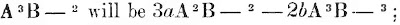and so on.CASE 1. Any rectangle, as AB, augmented by a perpetual flux, when, as yet, there wanted of the sides A and B half their momentswasorbut but as soon as the sides A and B are augmented by the other half moments, the rectangle becomesintoorFrom this rectangle subduct the former rectangle, and there will remain the excess aB + bA. Therefore with the whole increments a and b of the sides, the increment aB +bA of the rectangle is generated. Q.E.D. From: http://www.maths.tcd.ie/pub/HistMath/People/Newton/RouseBall/RB_Newton.htmlThe invention of the infinitesimal calculus was one of the great intellectual achievements of the seventeenth century. This method of analysis, expressed in the notation of fluxions and fluents, was used by Newton in or before 1666, but no account of it was published until 1693, though its general outline was known to his friends and pupils long anterior to that year, and no complete exposition of his methods was given before 1736.The idea of a fluxion or differential coefficient, as treated at this time, is simple. When two quantities - e.g. the radius of a sphere and its volume - are so related that a change in one causes a change in the other, the one is said to be a function of the other. The ratio of the rates at which they change is termed the differential coefficient or fluxion of the one with regard to the other, and the process by which this ratio is determined is known as differentiation. Knowing the differential coefficient and one set of corresponding values of the two quantities, it is possible by summation to determine the relation between them, as Cavalieri and others had shewn; but often the process is difficult, if, however, we can reverse the process of differentiation we can obtain this result directly. This process of reversal is termed integration. It was at once seen that problems connected with the quadrature of curves, and the determination of volumes (which were soluble by summation, as had been shewn by the employment of indivisibles), were reducible to integration. In mechanics also, by integration, velocities could be deduced from known accelerations, and distances traversed from known velocities. In short, wherever things change according to known laws, here was a possible method of finding the relation between them. It is true that, when we try to express observed phenomena in the language of the calculus, we usually obtain an equation involving the variables, and their differential coefficients - and possibly the solution may be beyond our powers. Even so, the method is often fruitful, and its use marked a real advance in thought and power.I proceed to describe somewhat fully Newton's methods as described by Colson.From Walter William Rouse Ball: A Short Account of the History of Mathematics, Published by Macmillan, 1901:The second part of this appendix to the Optics contains a description of Newton's method of fluxions. This is best considered in connection with Newton's manuscript on the same subject which was published by John Colson in 1736, and of which it is a summary.The fluxional calculus is one form of the infinitesimal calculus expressed in a certain notation, just as the differential calculus is another aspect of the same calculus expressed in a different notation. Newton assumed that all geometrical magnitudes might be conceived as generated by continuous motion; thus a line may be considered as generated by the motion of a point, a surface by that of a line, a solid by that of a surface, a plane angle by the rotation of a line, and so on. The quantity thus generated was defined by him as the fluent or flowing quantity. The velocity of the moving magnitude was defined as the fluxion of the fluent. This seems to be the earliest definite recognition of the idea of a continuous function, though it had been foreshadowed in some of Napier's papers.Newton's treatment of the subject is as follows. There are two kinds of problems. The object of the first is to find the fluxion of a given quantity, or more generally "the relation of the fluents being given, to find the relation of their fluxions.'' This is equivalent to differentiation. The object of the second or inverse method of fluxions is from the fluxion or some relations involving it to determine the fluent, or more generally "an equation being proposed exhibiting the relation of the fluxions of quantities, to find the relations of those quantities, or fluents, to one another.'' This is equivalent either to integration which Newton termed the method of quadrature, or to the solution of a differential equation which was called by Newton the inverse method of tangents. The methods for solving these problems are discussed at considerable length.Newton then went on to apply these results to questions connected with the maxima and minima of quantities, the method of drawing tangents to curves, and the curvature of curves (namely, the determination of the centre of curvature, the radius of curvature, and the rate at which the radius of curvature increases). He next considered the quadrature of curves and the rectification of curves. In finding the maximum and minimum of functions of one variable we regard the change of sign of the difference between two consecutive values of the function as the true criterion; but his argument is that when a quantity increasing has attained its maximum it can have no further increment, or when decreasing it has attained its minimum it can have no further decrement; consequently the fluxion must be equal to nothing.It has been remarked that neither Newton nor Leibnitz produced a calculus, that is, a classified collection of rules; and that the problems they discussed were treated from first principles. That, no doubt, is the usual sequence in the history of such discoveries, though the fact is frequently forgotten by subsequent writers. In this case I think the statement, so far as Newton's treatment of the differential or fluxional part of the calculus is concerned, is incorrect, as the foregoing account sufficiently shews.If a flowing quantity or fluent were represented by x, Newton denoted its fluxion by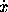, the fluxion ofor second fluxion of x by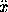, and so on. Similarly the fluent of x was denoted by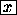, or sometimes by x' or [x]. The infinitely small part by which a fluent such as x increased in a small interval of time measured by o was called the moment of the fluent; and its value was shewn to beo. Newton adds the important remark that thus we may in any problem neglect the terms multiplied by the second and higher powers of o, and we may always find an equation between the co-ordinates x, y of a point on a curve and their fluxions,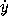. It is an application of this principle which constitutes one of the chief values of the calculus; for if we desire to find the effect produced by several causes on a system, then, if we can find the effect produced by each cause when acting alone in a very small time, the total effect produced in that time will be equal to the sum of the separate effects. I should here note the fact that Vince and other English writers in the eighteenth century usedto denote the increment of x and not the velocity with which it increased; that isin their writings stands for what Newton would have expressed byo   and what Leibnitz would have written as dx.I need not discuss in detail the manner in which Newton treated the problems above mentioned. I will only add that, in spite of the form of his definition, the introduction into geometry of the idea of time was evaded by supposing that some quantity (ex. gr. the abscissa of a point on a curve) increased equably; and the required results then depend on the rate at which other quantities (ex. gr. the ordinate or radius of curvature) increase relatively to the one so chosen. The fluent so chosen is what we now call the independent variable; its fluxion was termed the "principal fluxion''; and, of course, if it were denoted by x, thenwas constant, and consequently= 0.